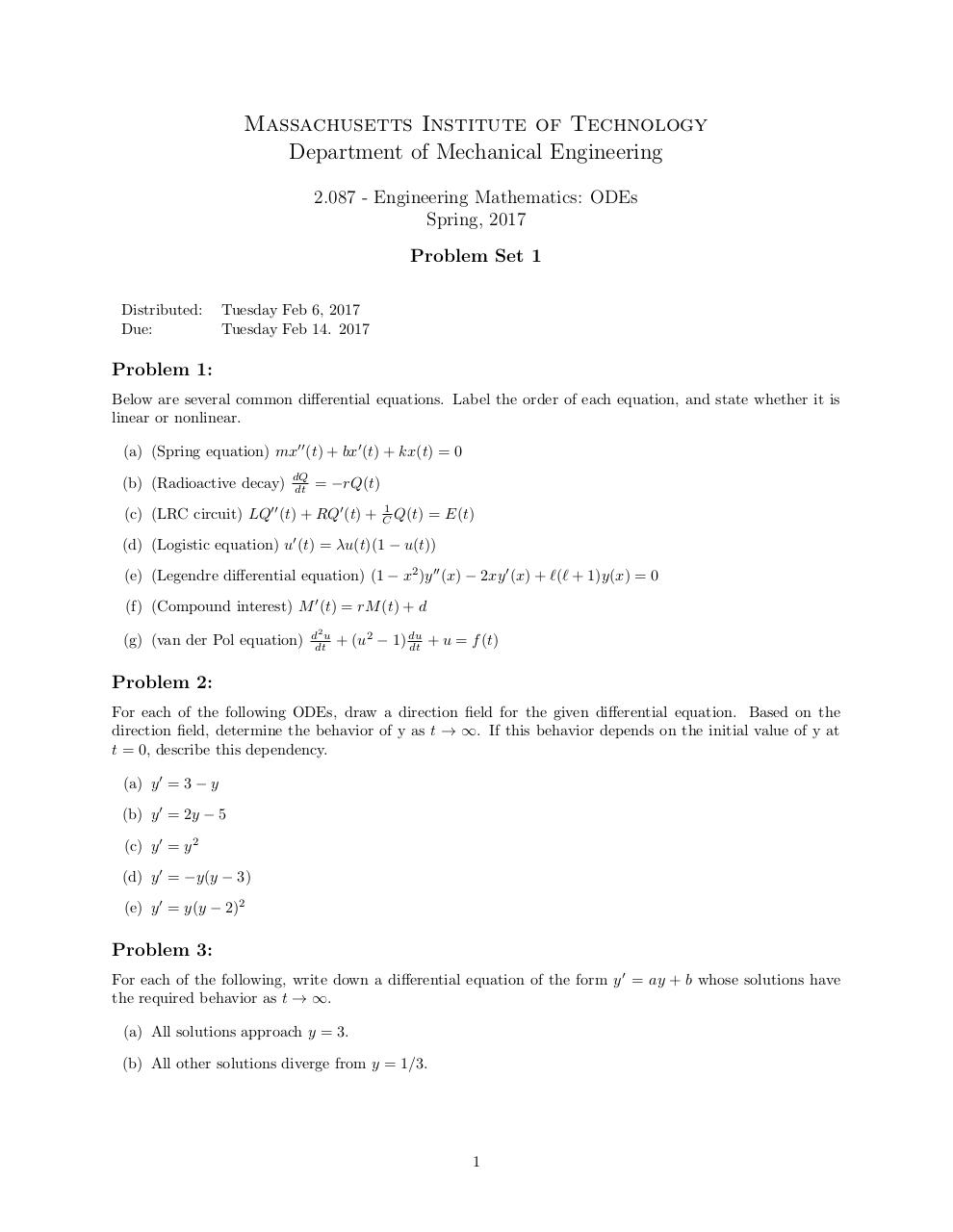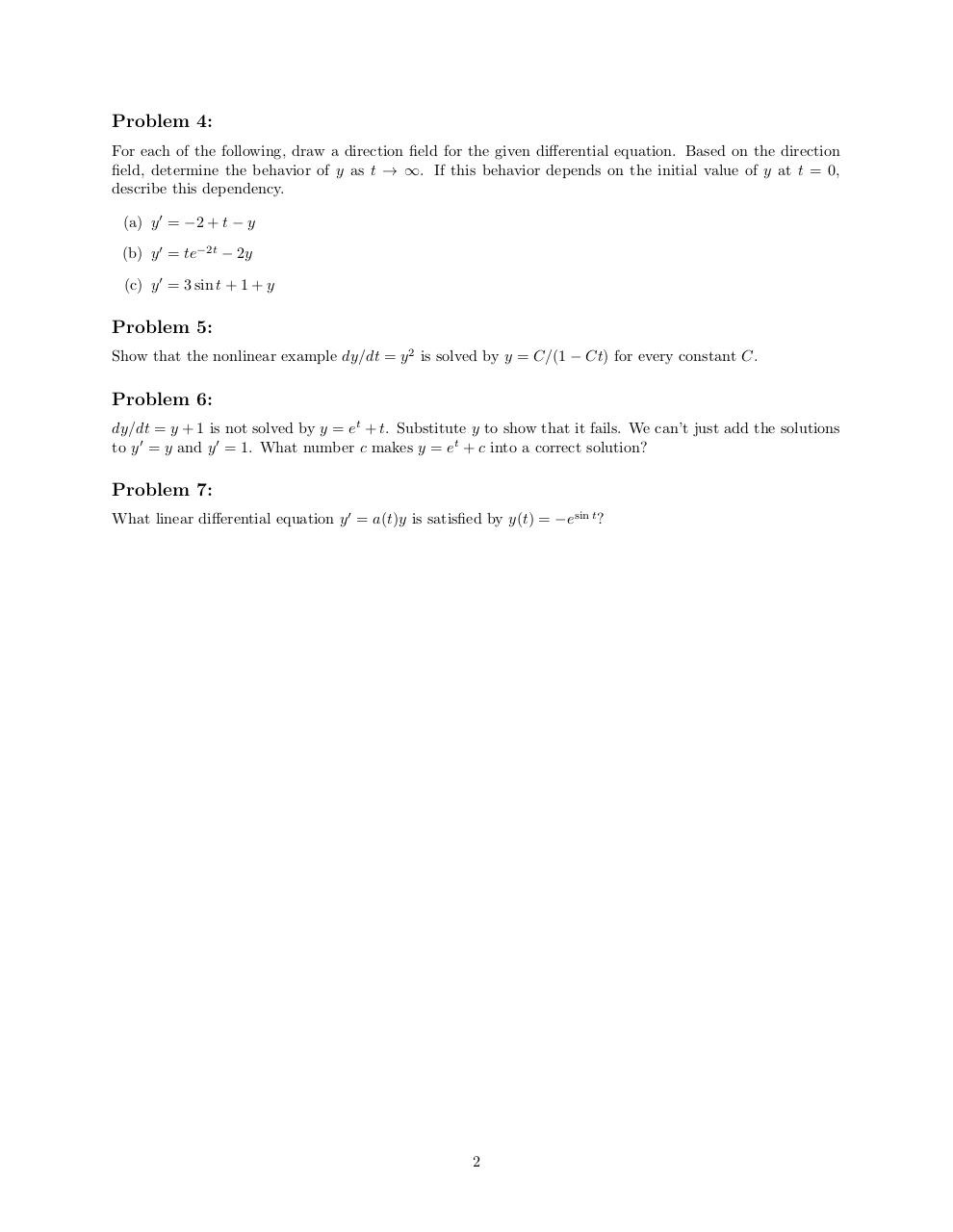# pset1 .pdf

### File information

Original filename: pset1.pdf

This PDF 1.5 document has been generated by TeX / MiKTeX pdfTeX-1.40.17, and has been sent on pdf-archive.com on 10/02/2017 at 04:23, from IP address 18.111.x.x. The current document download page has been viewed 314 times.
File size: 112 KB (2 pages).
Privacy: public file

pset1.pdf (PDF, 112 KB)

### Document preview

Massachusetts Institute of Technology
Department of Mechanical Engineering
2.087 - Engineering Mathematics: ODEs
Spring, 2017
Problem Set 1
Distributed:
Due:

Tuesday Feb 6, 2017
Tuesday Feb 14. 2017

Problem 1:
Below are several common differential equations. Label the order of each equation, and state whether it is
linear or nonlinear.
(a) (Spring equation) mx00 (t) + bx0 (t) + kx(t) = 0

dQ
dt

= −rQ(t)

(c) (LRC circuit) LQ00 (t) + RQ0 (t) +

1
C Q(t)

= E(t)

(d) (Logistic equation) u0 (t) = λu(t)(1 − u(t))
(e) (Legendre differential equation) (1 − x2 )y 00 (x) − 2xy 0 (x) + `(` + 1)y(x) = 0
(f) (Compound interest) M 0 (t) = rM (t) + d
(g) (van der Pol equation)

d2 u
dt

+ (u2 − 1) du
dt + u = f (t)

Problem 2:
For each of the following ODEs, draw a direction field for the given differential equation. Based on the
direction field, determine the behavior of y as t → ∞. If this behavior depends on the initial value of y at
t = 0, describe this dependency.
(a) y 0 = 3 − y
(b) y 0 = 2y − 5
(c) y 0 = y 2
(d) y 0 = −y(y − 3)
(e) y 0 = y(y − 2)2

Problem 3:
For each of the following, write down a differential equation of the form y 0 = ay + b whose solutions have
the required behavior as t → ∞.
(a) All solutions approach y = 3.
(b) All other solutions diverge from y = 1/3.

1

Problem 4:
For each of the following, draw a direction field for the given differential equation. Based on the direction
field, determine the behavior of y as t → ∞. If this behavior depends on the initial value of y at t = 0,
describe this dependency.
(a) y 0 = −2 + t − y
(b) y 0 = te−2t − 2y
(c) y 0 = 3 sin t + 1 + y

Problem 5:
Show that the nonlinear example dy/dt = y 2 is solved by y = C/(1 − Ct) for every constant C.

Problem 6:
dy/dt = y + 1 is not solved by y = et + t. Substitute y to show that it fails. We can’t just add the solutions
to y 0 = y and y 0 = 1. What number c makes y = et + c into a correct solution?

Problem 7:
What linear differential equation y 0 = a(t)y is satisfied by y(t) = −esin t ?

2#### HTML Code

Copy the following HTML code to share your document on a Website or Blog

#### QR Code### Related keywords